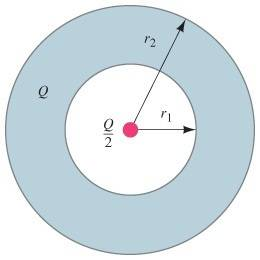# Electric Potential and a Conductor

## Homework Statement

A hollow spherical conductor, carrying a net charge +Q, has inner radius r1 and outer r2=2r1 radius (see the figure ). At the center of the sphere is a point charge +Q/2.A.Write the electric field strength E in all three regions as a function of r: for r>r2,r1<r<r2, and .0<r<r1

B. Determine the potential as a function of r , the distance from the center, for r>r2. Take V=0 at r=∞.

C. Determine the potential as a function of r for r1<r<r2.

D.Determine the potential as a function of r for 0<r<r1.

v=k(q/r)
u=vq

## The Attempt at a Solution

I have solved A and B.

A. $$E_{r>r_2}=\frac{1}{4\pi\epsilon_0}(\frac{3Q}{2r^2})$$
$$E_{r_1<r<r_2}=0$$
$$E_{0<r<r_1}=\frac{1}{4\pi\epsilon_0}(\frac{Q}{2r^2})$$

B. $$V=\frac{1}{4\pi\epsilon_0}(\frac{3Q}{2r})$$

I can't figure out how to solve C or D.

For C I think the charge depends on how far into the conductor you want r to be. Since the charge is distributed over the conductor. Can someone guide my thinking?

BruceW
Homework Helper
You've got the electric field everywhere, and so you can find the electric potential anywhere (keeping in mind that the electric potential varies continuously). You know the equation that links the electric field and the electric potential?

Yes, I know that

$$vec(E)=-vec(\nabla V)$$

I also know that

$$E=-\frac{dV}{ds}$$ when ds||E

If I use the second equation I set E equal to zero and you have v=0 which is wrong. The first one I'm not even sure how to use since I have magnitudes of vectors not vectors.

Never mind, what that tells me is that the electric potential inside the conductor is constant, but how do I derive the value?

BruceW
Homework Helper
Take V=0 at r=∞.

You've already used this to find the potential outside the conductor (part b), and the potential varies continuously, so what does this tell you about the potential just outside and inside the conductor?

Hmm interesting. I've got it now. The electric potential inside the shell is the sum of the electric potential on the outer edge and the inner edge.

This yields: $$V=k\frac{3Q}{4r_1}$$

Ok, but I'm still confused on the next question. I would think the electric potential inside the cavity would be:

$$V=k\frac{Q}{2r}$$

However, the software is telling me I am wrong. Am I missing something?

BruceW
Homework Helper
Hmm interesting. I've got it now. The electric potential inside the shell is the sum of the electric potential on the outer edge and the inner edge.

That's not right. The electric potential inside the conductor is constant, and there are no 'jumps' (i.e. discontinuities) of the potential at the edges. And you know the potential just outside, so what will be the potential inside the conductor, and on the inside edge?

BruceW
Homework Helper
$$V=k\frac{3Q}{4r_1}$$
This is right though (for the potential inside the conductor). Maybe I just didn't understand how you worded it. You said that "The electric potential inside the shell is the sum of the electric potential on the outer edge and the inner edge." But the potential inside the shell is equal to the potential on the outer edge (and also equal to the potential on the inner edge).

BruceW
Homework Helper
Ok, but I'm still confused on the next question. I would think the electric potential inside the cavity would be:

$$V=k\frac{Q}{2r}$$

However, the software is telling me I am wrong. Am I missing something?

You're close. You have the bit that varies with radius. But there is another term. You can find it directly by integration. Or you can guess it, since you know what the potential must be at r=r1.